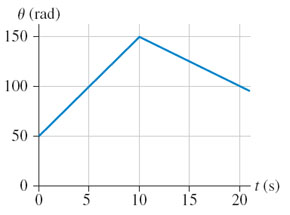# Problem: (Figure 1) shows the angular position of a potter's wheel.Part AWhat is the angular displacement of the wheel between t = 5 s and t = 15 s?Express your answer using two significant figures.Part BWhat is the angular velocity of the wheel at 5 s?Express your answer using two significant figures.

###### FREE Expert Solution

Part A

Between t = 5 s and t = 15 s:

86% (238 ratings)###### Problem Details

(Figure 1) shows the angular position of a potter's wheel.Part A

What is the angular displacement of the wheel between t = 5 s and t = 15 s?

Part B

What is the angular velocity of the wheel at 5 s?